Return to Free Library

Return to Number Menu

We will now discuss one important aspect of the hexagon – that of tessellations.  We will expand from just the hexagon to cover 2D and 3D tessellations and geometric tiling.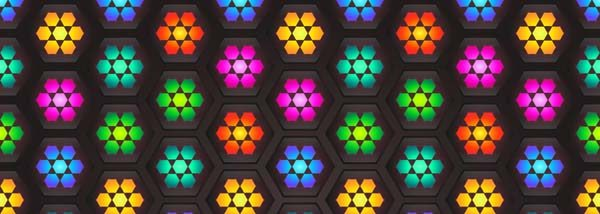We will start with one important quality of the hexagon – that of hexagonal packing.

# Hexagonal Packing

Circles, spheres & cylinders fill space more efficiently in a hexagonal pattern than a square pattern.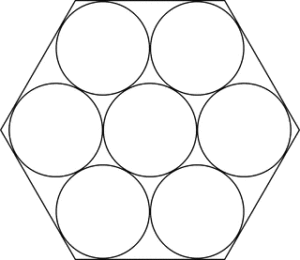Circular packing in a hexagonal pattern.  Curved triangular gaps remain.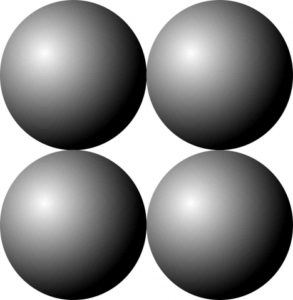Circular packing in a square pattern.  Larger curved diamond shaped gaps remain.

When circles fill space in a hexagonal pattern, they leave curved triangular gaps between them.

When the circles are converted to hexagons, no gaps are left at all.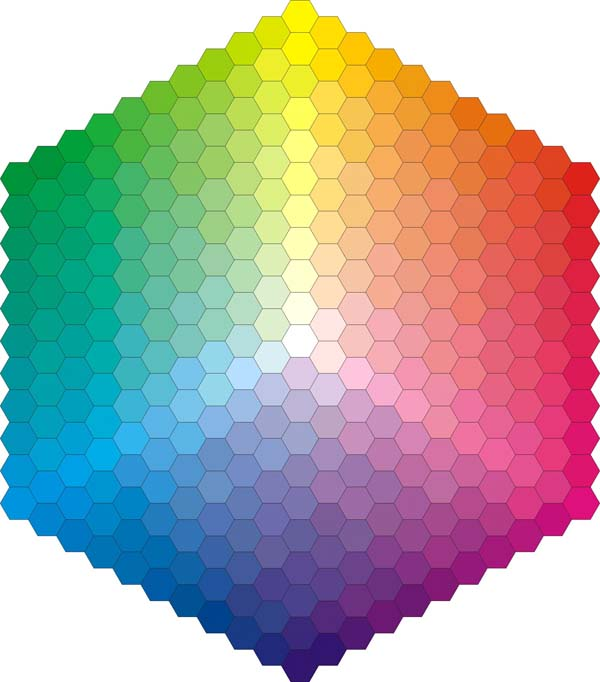“Hexagonal tessellation extends the circle’s principle of equality in all directions in a balanced sharing of space, materials, time, and energy.  It creates three-corner 120-degree joints, which provide the triangle’s strength and structural excellence to the package.”1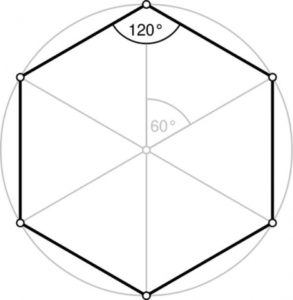# Symmetry – ‘Measuring Together’

David Wade tells us, “Any notion of symmetry is completely entangled with that of asymmetry; we can scarcely conceive of the former without invoking thoughts of the latter.  Symmetry precepts are always involved with categorization, with classification and observed regularities; in short, with limits.  But in itself symmetry is unlimited; there is nowhere that its principles do not penetrate.  In addition, symmetry principles are characterized by a quietude, a stillness that is somehow beyond the bustling world; yet, in one way or another, they are almost always involved with transformation, disturbance, or movement.”2

Symmetrical manipulations are as follows:

Displacement (translation) – movement from place or position a certain distance in a certain direction – leaves it appearing ‘the same’.

Reflection – inverting a tile with respect to a plane, each element being transferred perpendicularly through the plane to a point the same distance the other side of it.

Rotation – the action of rotating a tile around an axis or center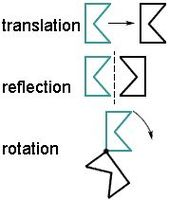Spiraling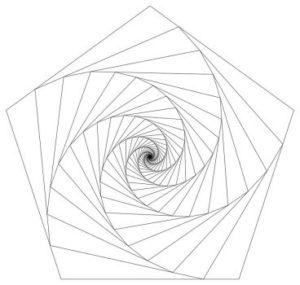Scaling – keeping the same shape but changing the relative size of a tile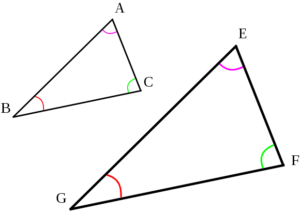Stretching – making the tile longer or wider without tearing or breaking

Folding

Various combinations of the above can be used.

Some of these manipulations can be seen in dual uniform tilings, such as displacement, reflection and roration.  In these cases the same shape is used.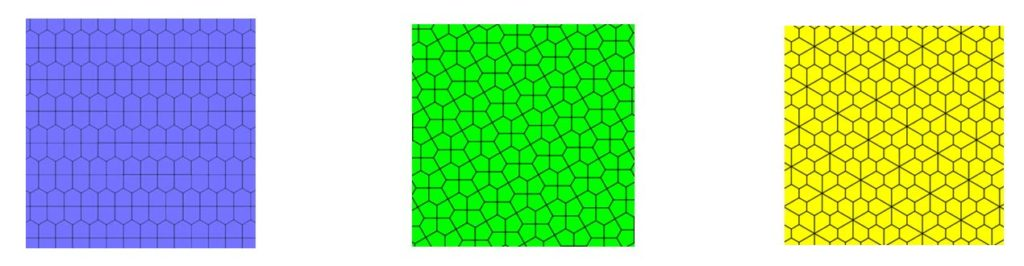# Tessellations

Tessellation occurs when the same regular polygon is used to fill the plane, leaving no gaps, nor overlapping.

There are regular tilings with regular polygonal tiles of all the same shape.

There are also semiregular tilings with regular tiles of more than one shape and with every corner identically arranged.

A tiling that lacks a repeating pattern is called ‘non-periodic’.  We have seen these with Penrose pentagonal tilings in Article 54.

The part that repeats is called a ’tile’ regardless of its shape.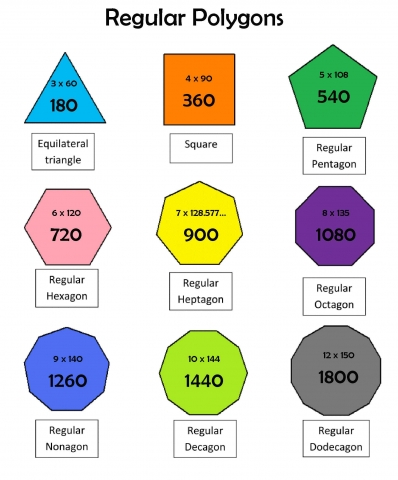“When the tessellation is made of regular polygons, the most common notation is the vertex configuration, which is simply a list of the number of sides of the polygons around a vertex.

To name a tessellation, go around a vertex (corner point) and write down how many sides each polygon has, in order.

For example, a square tiling would be 4.4.4.4.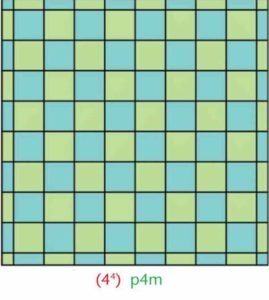A regular hexagonal tiling would be 6.6.6.” 3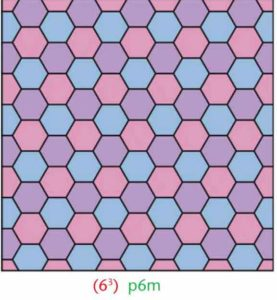A semiregular tiling of hexagons and triangles (snub hexagonal tiling) would be 3.3.3.3.6.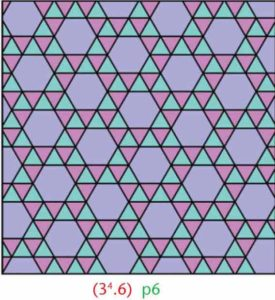When naming, always start with the polygon with the least number of sides.

# Schlafli Symbol Notation

Schlafli symbols are a more compact way to describe tilings.

For instance, an equilateral triangle is {3}.  A Square is {4}.  A regular pentagon is {5}.

Below is a chart with convex and star polygons with their Schlafli symbols.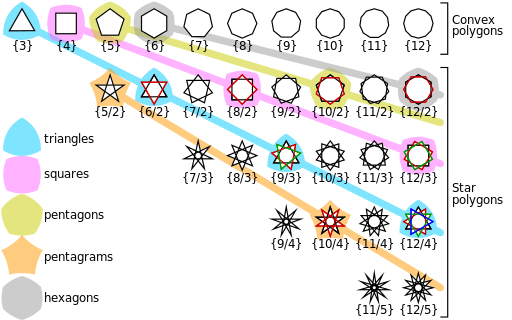The Platonic solids also have Schlafli Symbols.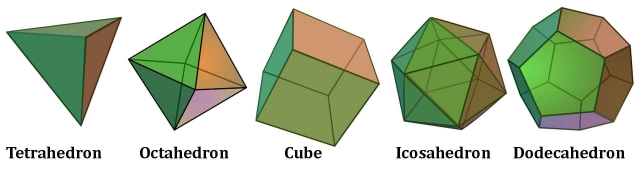They are as follows:

• Tetrahedron {3, 3}
• Octahedron {3, 4}
• Cube {4, 3}
• Icosahedron {3, 5}
• Dodecahedron {5, 3}

The tetrahedron is {3, 3} because each polygon face has 3 sides.  Plus, at each vertex 3 polygons meet.  Hence the symbol is {3, 3}.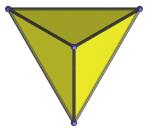The Icosahedron has 3-sided polygon faces like the tetrahedron (equilateral triangles).  At each vertex 5 triangles come together.  Hence the symbol is {3, 5}.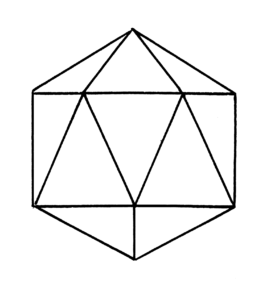Note that the reversal of the symbol gives the dual polyhedron.

Recall, the octahedron and cube are duals.  The icosahedron and dodecahedron are duals; and the tetrahedron is dual with itself.

The subject of symmetry and Schlafli symbols is a large one.  We have only introduced it here.

# Regular Tilings

Only three regular polygon tessellations are possible:

• Triangle {3, 6}
• Square {4, 4}
• Hexagon {6, 3}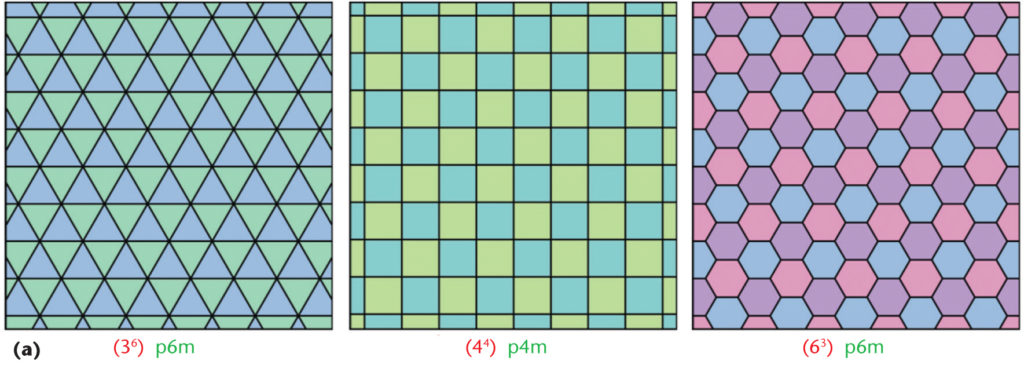# Semi-regular Tiling

Semi-regular tiling allows for more than one type of polygon.

Each vertex is the same.

There are 8 total possible (pictured below):

• triangle/hexagon – 2 versions {3, 6, 3, 6}      and      {3, 3, 3, 3, 6}
• octagon/square {4, 8, 8}
• triangle/square – 2 versions {3, 3, 3, 4, 4} and      {3, 4, 3, 3, 4}
• triangle/dodecagon {12, 3, 3}
• hexagon/square/triangle {3, 4, 6, 4}
• hexagon/square/dodecagon {4, 6, 12}
• Dodecagons can be filled in further as they are composed of hexagons, triangles and squares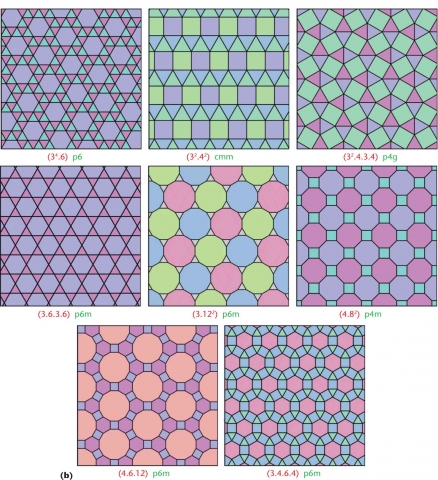Pentagons can only be tiled in 3D.  Look at the image below.  If you cut out the white triangles and fold this together the pentagons will tessellate.  This will form 1/2 of a dodecahedron.  Thus, pentagons can only tile in 3D.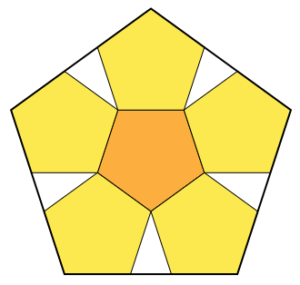Heptagons {7} and nonagons {9} cannot be tiled in 2D or 3D.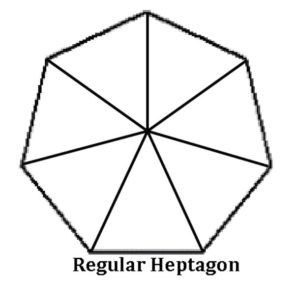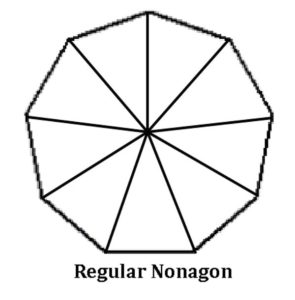# Demi-regular Tilings

There are 20 total.  These are seen below.

They allow for more than 1 polygon and for 2 vertex situations.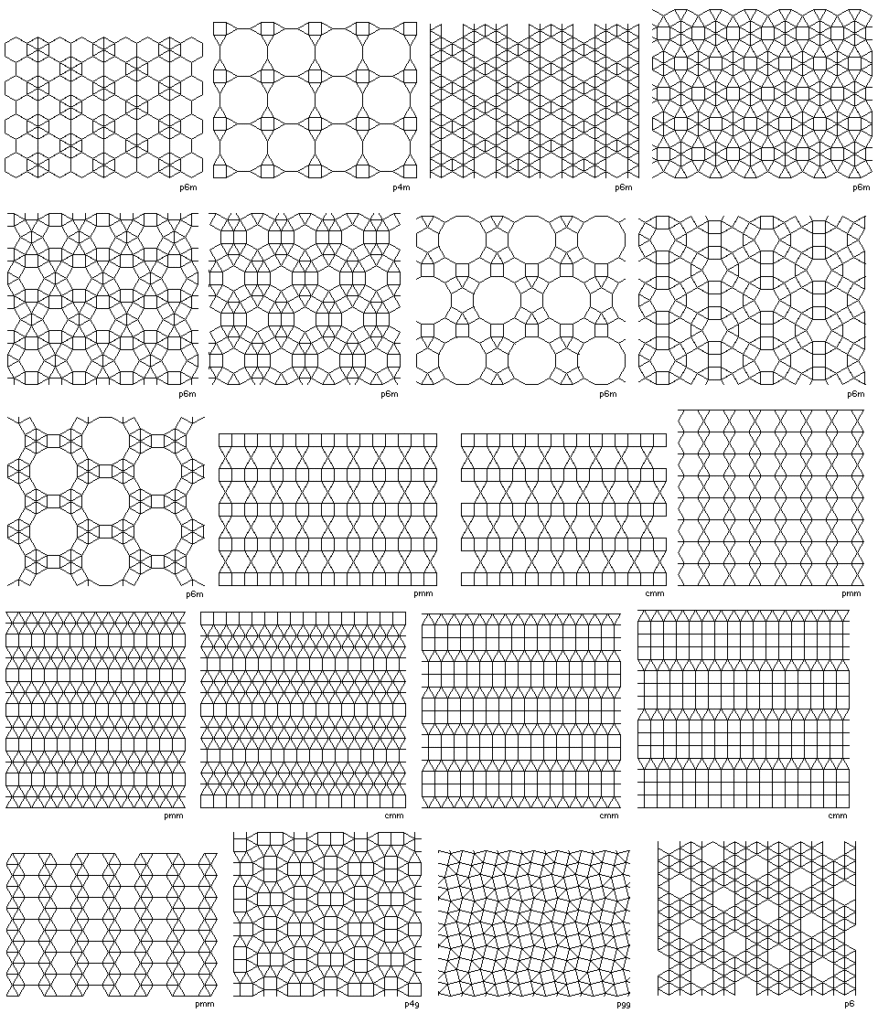These tilings are the basis for Celtic & Islamic art.

These tilings also appear in crystal, molecular and cellular structures in nature.  This is key!  At the molecular structure of all matter exists beautifully ordered geometric lattices upon which life is built.  All physical matter is composed of geometry within geometry – a fractal-holographic matrix of interconnected consciousness and information.

# 3D Tilings

There are three polyhedra that tile 3D space:

Cube (Platonic solid)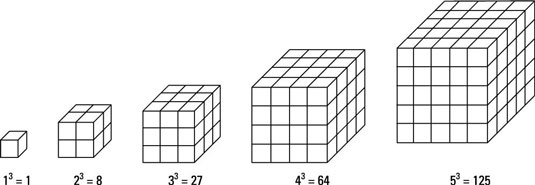Rhombic dodecahedron (Catalan solid)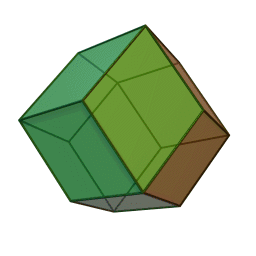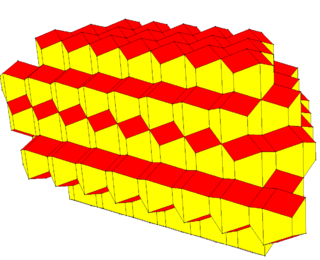Truncated octahedron (Archimedean solid)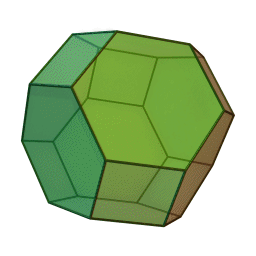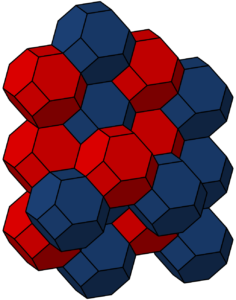There are also Quasiregular 3D tessellations that use more than 1 polyhedron.  There are many of these.  Three examples are pictured below:

Regular octahedra and tetrahedra (2Platonic solids)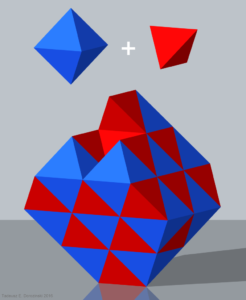Small rhombicuboctahedron, cuboctahedron and cube (2Archimedean solids & 1 Platonic solid)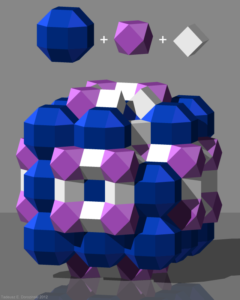Truncated Cubes, Rhombcuboctahedra, Cubes, and Octagonal Prisms (2 Archimedean solids, 1 Platonic solid & 1 prism)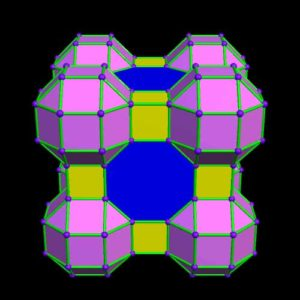Visit RobertLovesPi.net for amazing visuals of polyhedra, tessellations and more.

# Pentagonal Tilings

In Article 54 we covered pentagonal tilings and quasi-crystals.  We will briefly review pentagonal tilings now.

There are 15 known types of pentagons that tile a plane.

Here are the fifteen types: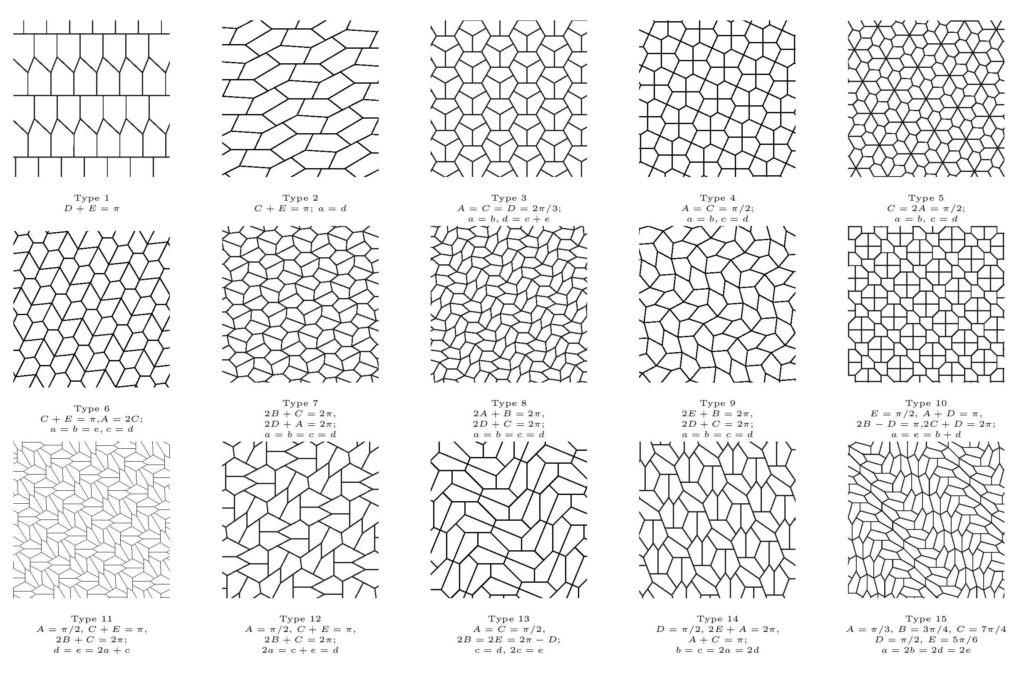There are three main types of non-periodic pentagonal Penrose tilings.  These are P1, P2, and P3.

P1 – uses pentagons, pentagrams, a ‘boat’ (roughly 3/5 of a star) and a ‘diamond’ (a thin rhombus).

Pentagons – red, grey, dark blue

Pentagrams – green

Boats – light blue

Diamonds – yellow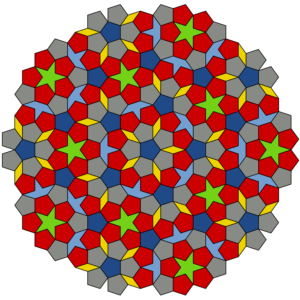P2 – uses the ‘kite’ and ‘dart’.

The kite (dark yellow) is a quadrilateral with 4 interior angles: 72°, 72°, 72° and 144°.

The dart (light yellow) is a non-convex quadrilateral with four interior angles: 36°, 72°, 36° and 216°.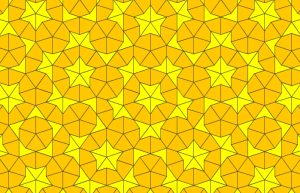P3 – uses a pair of rhombuses (rhombs).

The thin rhomb (light blue) has 4 corners with angles: 36°, 144°, 36° and 144°.

The thick rhomb (dark blue) has 4 angles: 72°, 108°, 72°, and 108°.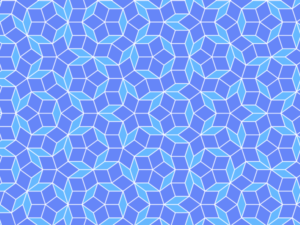# Voronoi Tessellations & Delaunay Triangulation

We first discussed Voronoi tessellations & Delaunay triangulation in Article 33.  We will review that here as the hexagonal structure that forms from it is extremely important in the structure/function/order of so many things found in nature.

“Voronoi tilings are tessellations where each tile is defined as the set of points closest to one of the points in a discrete set of defining points.  (Think of geographical regions where each region is defined as all the points closest to a given city or post office.) The Voronoi cell for each defining point is a convex polygon.  The Delaunay triangulation is a tessellation that is the dual graph of a Voronoi tessellation.  Delaunay triangulations are useful in numerical simulation, in part because among all possible triangulations of the defining points, Delaunay triangulations maximize the minimum of the angles formed by the edges.  Voronoi tilings with randomly placed points can be used to construct random tilings of the plane.”4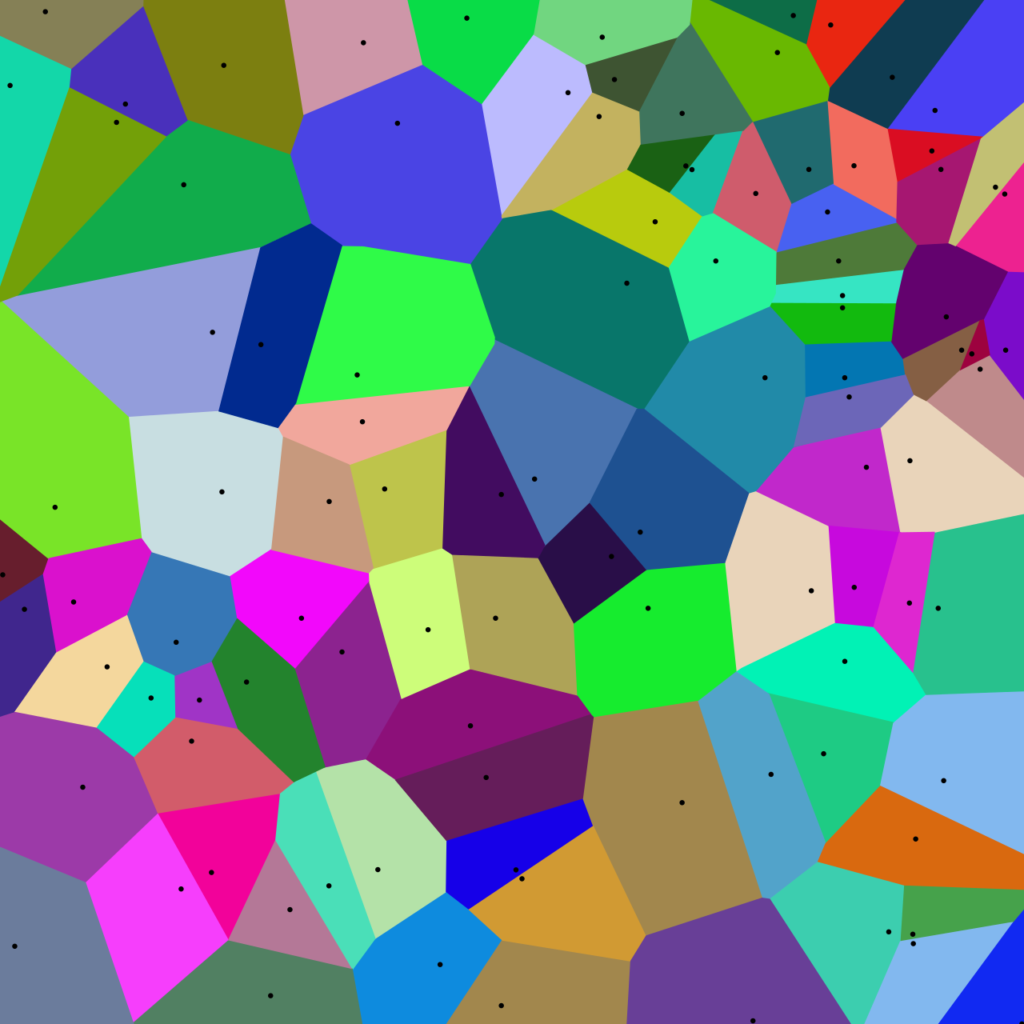As we show throughout Cosmic Core, Delaunay Triangulation and Voronoi Tessellations are a process that nature undergoes to form many structures in nature.  These include molecular structures and cellular structures of plant seeds, pollen, roots, leaves, flowers and fruits, as well as the cellular structure of fungi, insects and insect wings, and the cellular structure of many animal tissues.  We saw examples of these in the previous two articles on the Hexad.

In the article Nature by Numbers3 it says, Voronoi Tessellations “are based on a distribution pattern that is easily recognizable in many natural structures, like the wings of some insects and plant leaves.”

The article goes on to show how Voronoi tessellations and Delaunay triangulation can form the structure of a dragonfly wing.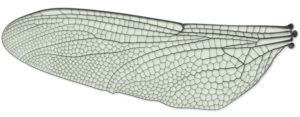Delaunay triangulation involves a set of discrete points on a plane.  Triangles are formed among the points so that no outside point falls in the circumcircle of the triangles.  Connecting the centers of the circumcircles produces the Voronoi tessellations that are hexagonal and pentagonal in structure.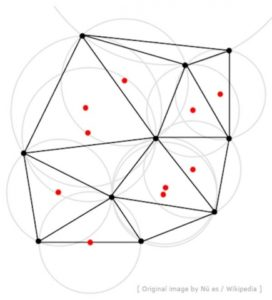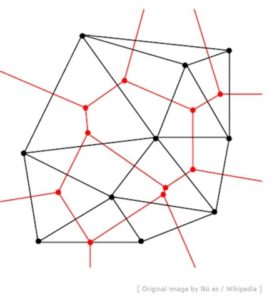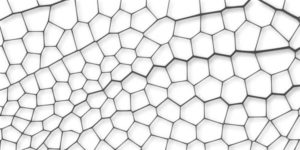Visit: http://www.scienceonthenet.eu/content/article/nature-numbers to see clearly what is going on here.

Delaunay triangulations maximize the minimum angle of all the angles of the triangles in the triangulation.  They tend to avoid sliver triangles (extremely acute triangles that look like slivers).

The moving image below shows how Voronoi tessellations interact with phyllotaxis to produce the beautiful geometry found in the seed heads of some flowers such as daisies and sunflowers.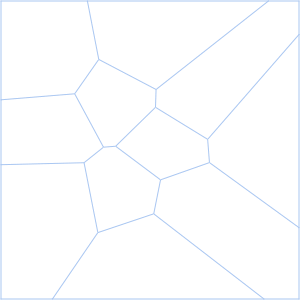# Hyperbolic Tiling

“The hyperbolic plane cannot be metrically represented in the Euclidean plane, but Poincare described ways that it can be conformally represented in the Euclidean plane.  One of those is to represent the hyperbolic plane as the points inside a disk.  For this representation, a straight line in the hyperbolic plane is represented as the part (in the disk) of a circle that meets the boundary of the disk at right angles.”5

The below images show hyperbolic tiling of a heptagon and a pentagonal star polygon.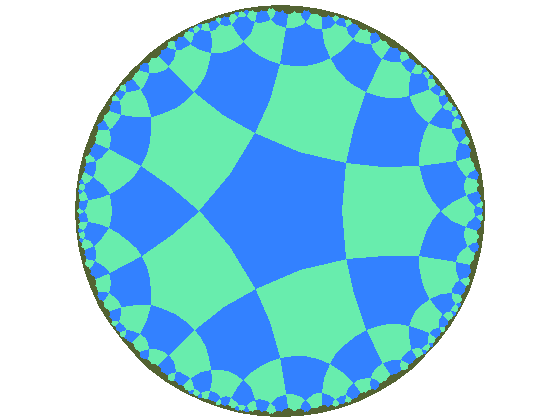There are infinitely many regular tessellations of the hyperbolic plane.  Yet you cannot put an infinite plane in a finite region without a lot of distortion.  That is why the lines look curved, when in actuality they are straight.  This is also why the center polygon looks larger and the circumference polygons looks smaller.

In hyperbolic tiling all vertices are congruent and the tiling has a high degree of rotational and translational symmetry.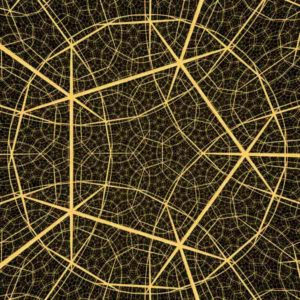A hyperbolic dodecahedron

# Tessellations in Art

The Sumerians (4000 BC) used tessellations in building wall decorations formed by patterns of clay tiles.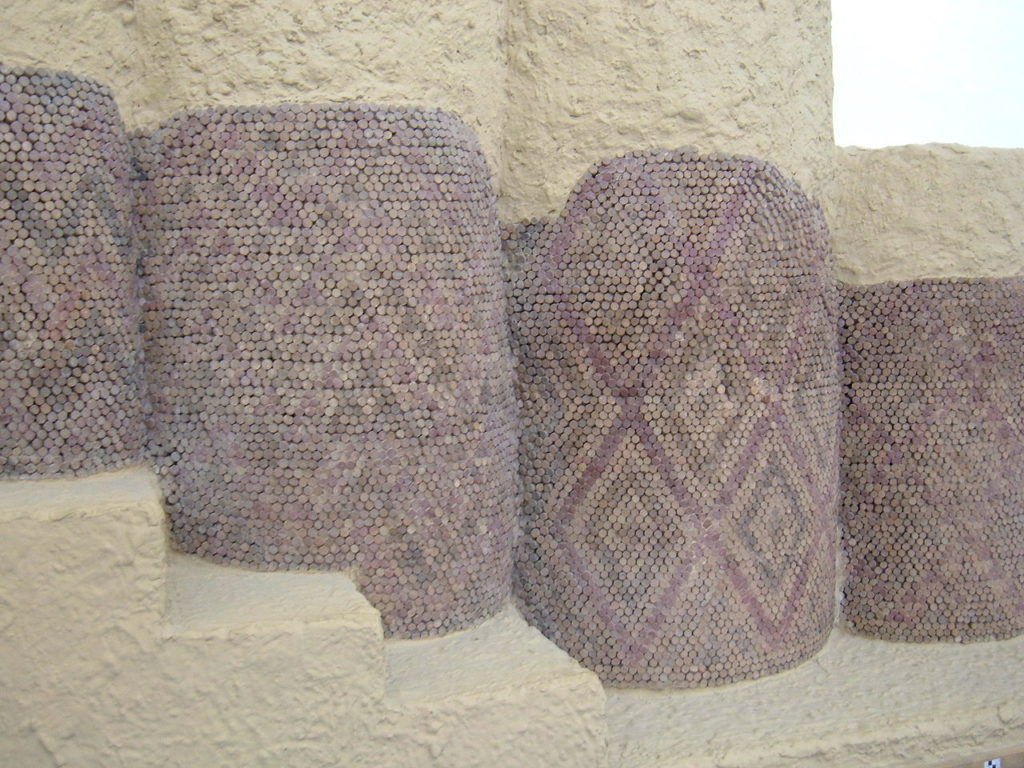The ancient Romans used tessellating patterns for mosaic floor panels of stone, tile and glass.  Here is one example from Syria, near Antioch, from the 2nd century AD.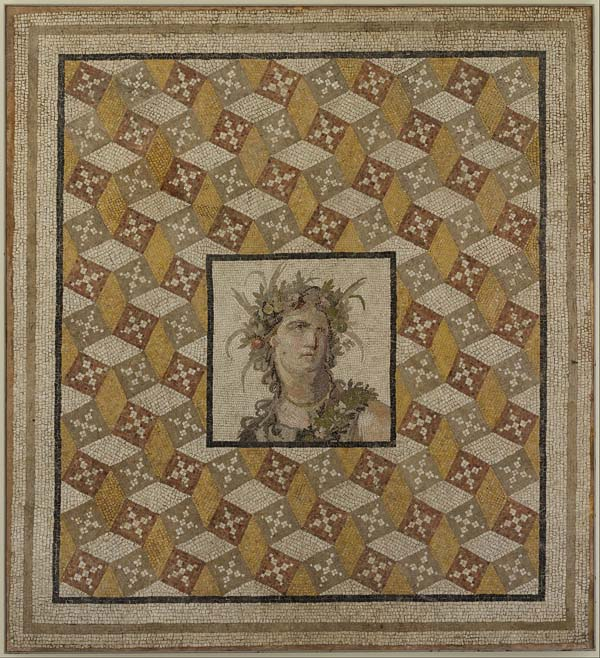Here is an ancient roman geometric mosaic from the “Palazzo Massimo alle Terme” National Roman Museum of Rome, Italy.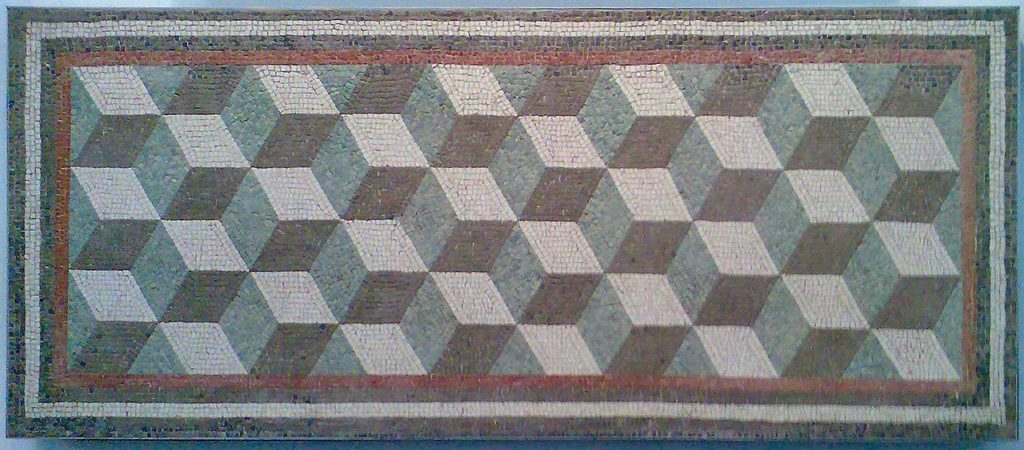Tessellations are very common in Islamic art and architecture.  The topic of Islamic tiling is a big one.  Here we offer only a few exquisite examples.  Many more can be found at the Alhambra in Granada, Spain and La Mezquita in Cordoba, Spain.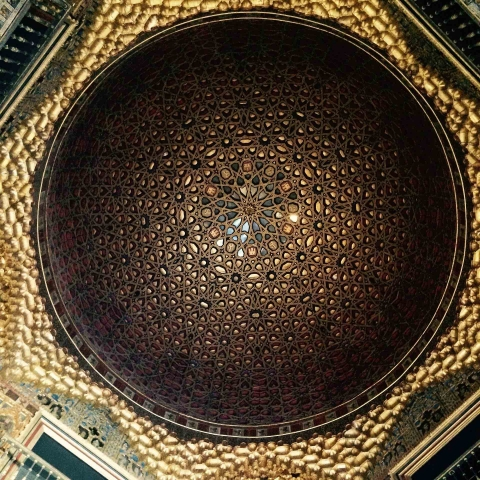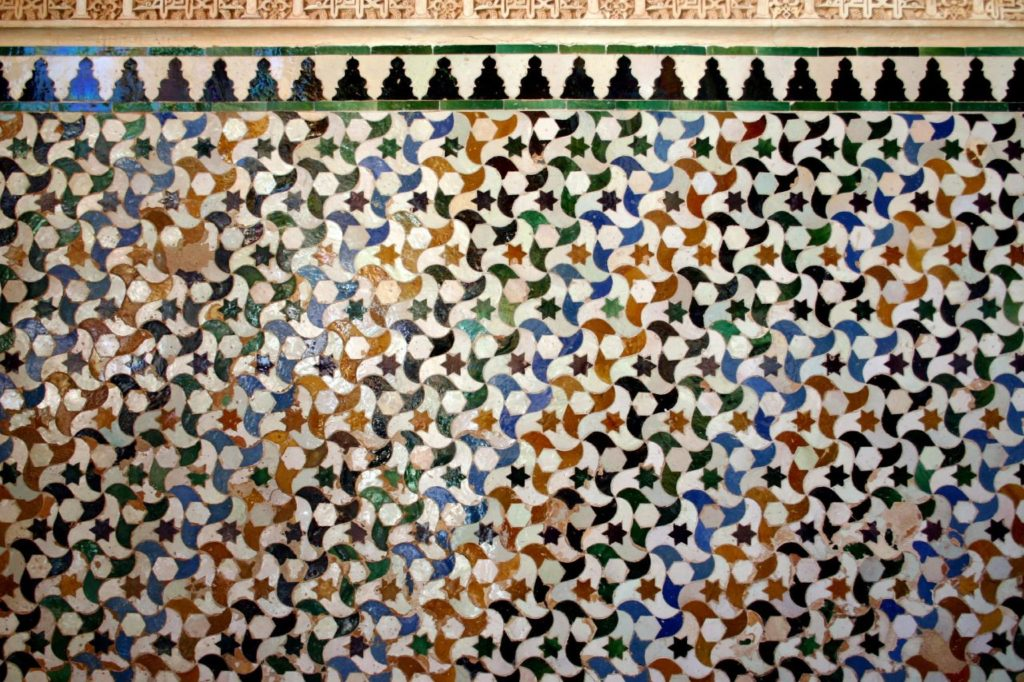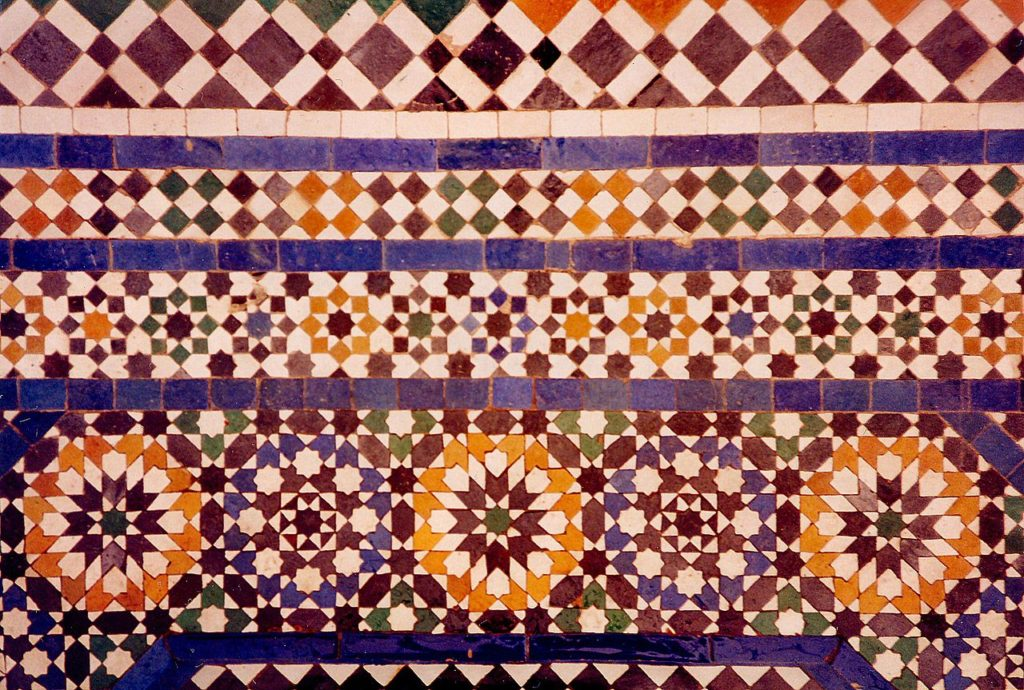Here are some other examples of Islamic tiling patterns.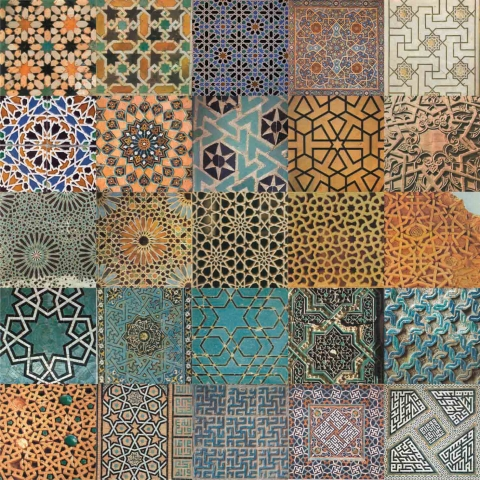Johannes Kepler, in 1619, wrote about regular and semiregular tessellations in Harmonices Mundi.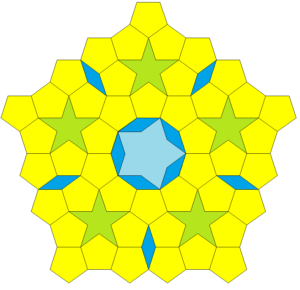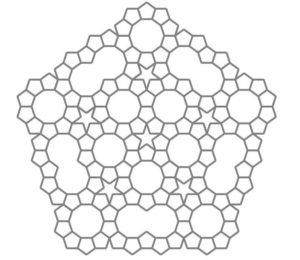Tessellations are found in quilting, weaving, origami and stained glass art.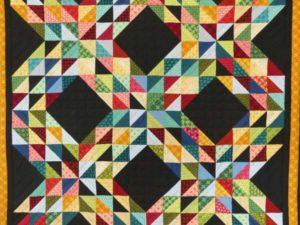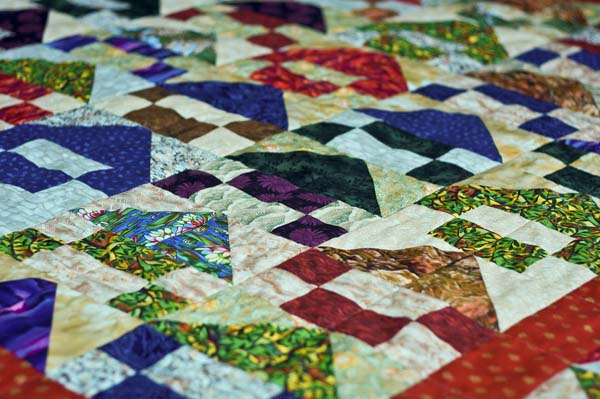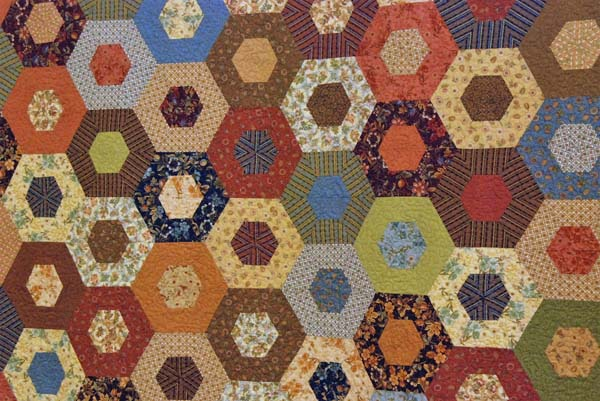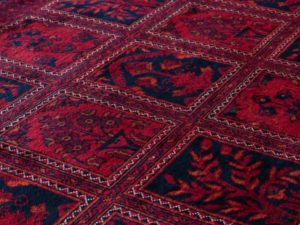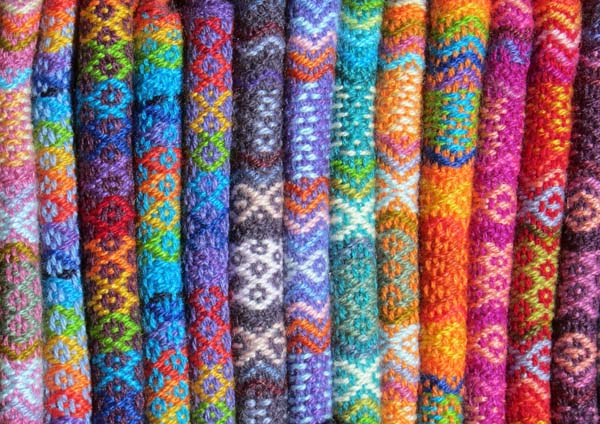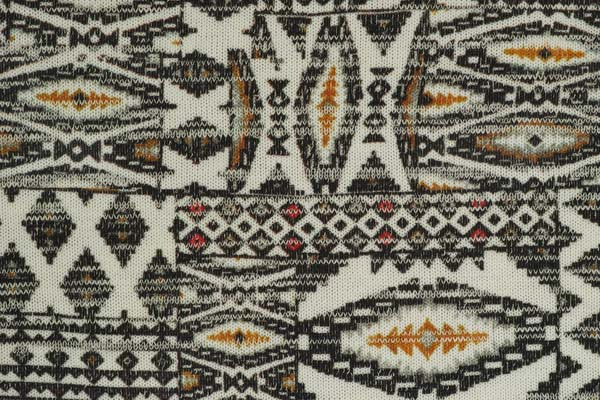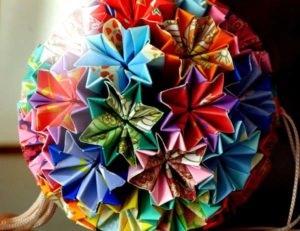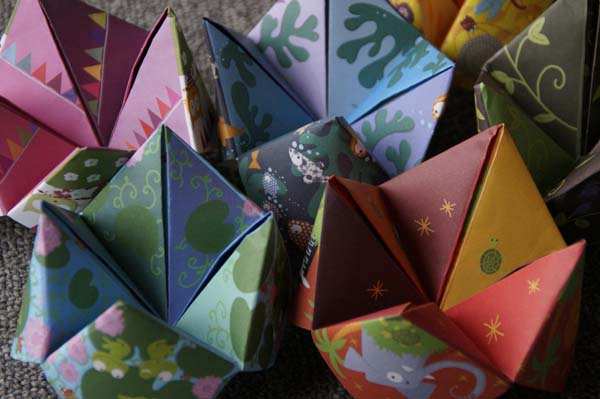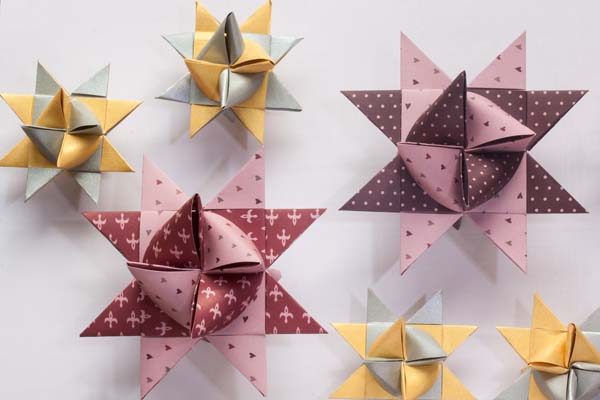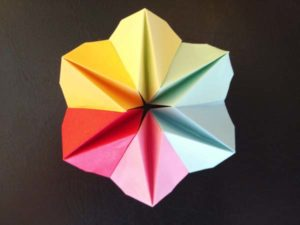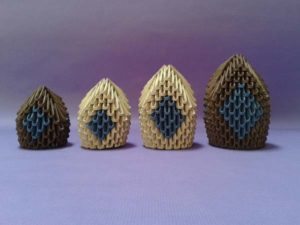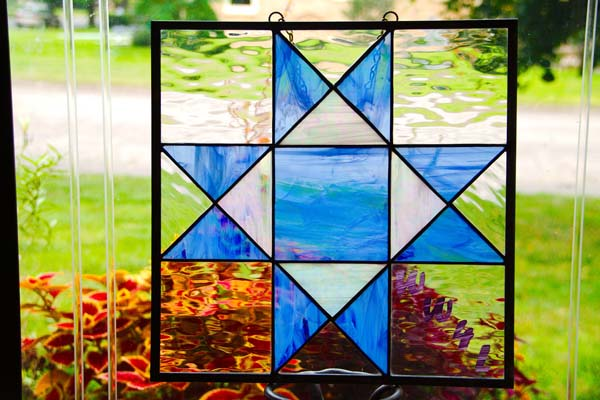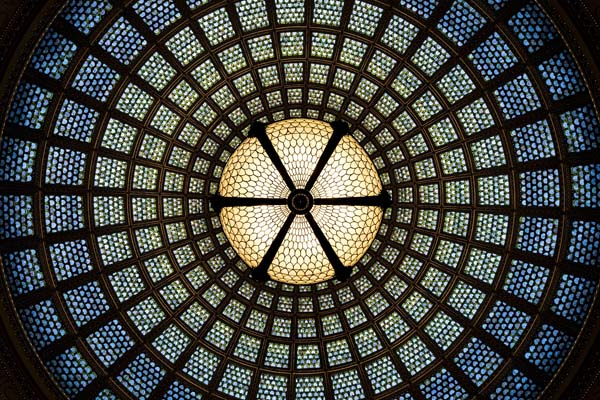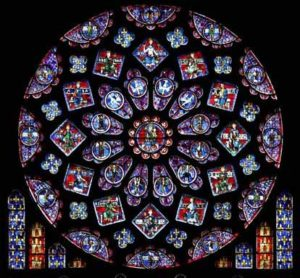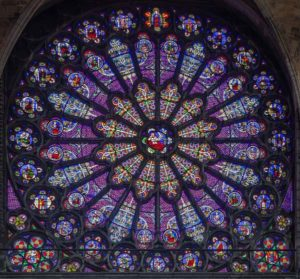And perhaps the best known artist that uses tessellations is M.C. Escher.

# M.C. Escher (1898-1972)

M.C. Escher is the master of tessellations, sometimes called the ‘Father’ of modern tessellations.  He was a Dutch graphic artist who made mathematically inspired woodcuts, lithographs, and mezzotints.

Escher’s “work features mathematical objects and operations including impossible objects, explorations of infinity, reflection, symmetry, perspective, truncated and stellated polyhedra, hyperbolic geometry and tessellations.”6

He applied knowledge of hexagonal tiling patterns with images from nature and fantasy to create intriguing designs.

He used both plane tessellations and hyperbolic tessellations.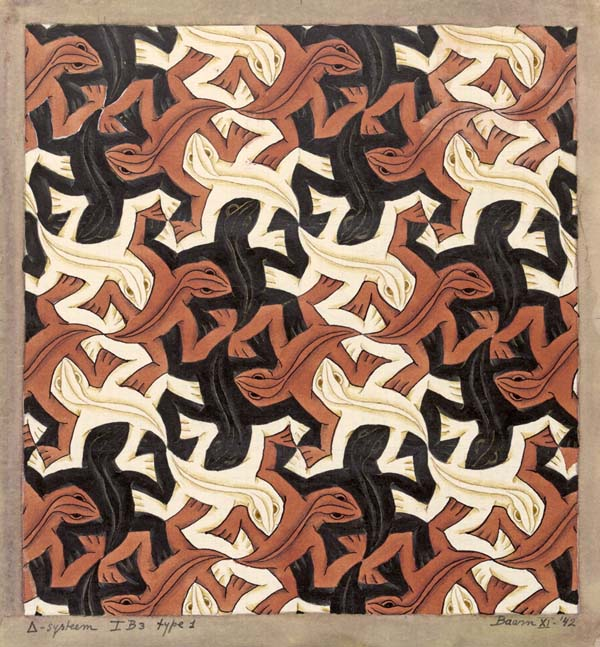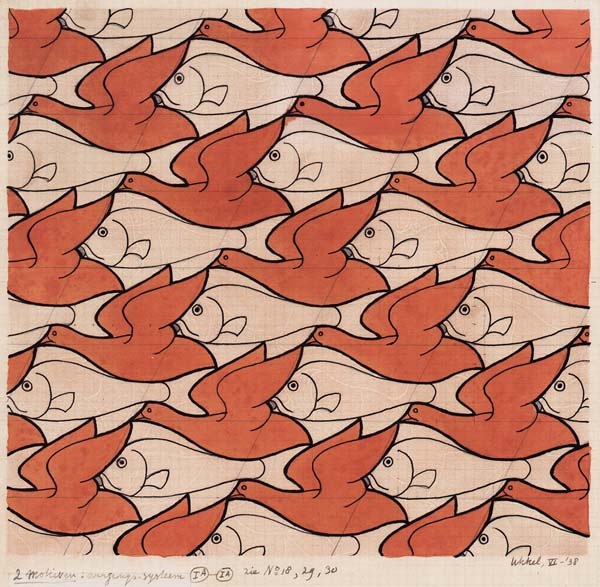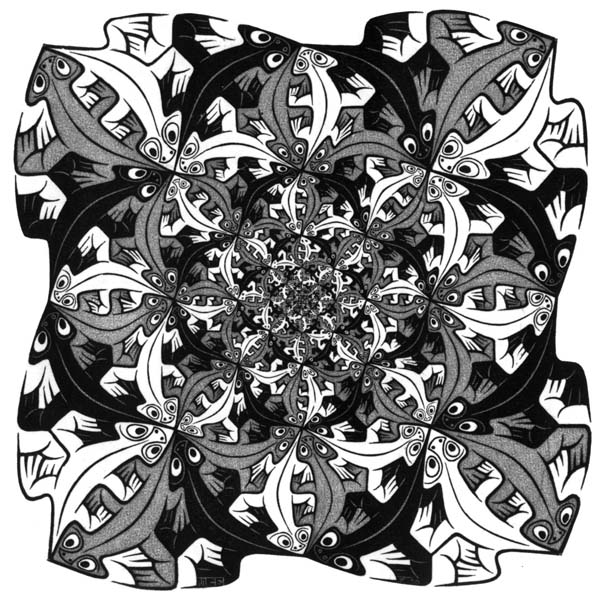Visit his official website here: http://www.mcescher.com/ to see the full catalog of his work.

1. Schneider, Michael, A Beginner’s Guide to Constructing the Universe, Harper Perennial, 1994
2. Wade, David, Symmetry: The Ordering Principle, Bloomsbury USA, 2006
3. https://en.wikipedia.org/wiki/Tessellation
4. http://www.scienceonthenet.eu/content/article/nature-numbers
5. Hyperbolic Tessellations, https://mathcs.clarku.edu/~djoyce/poincare/poincare.html
6. https://en.wikipedia.org/wiki/M._C._Escher

Return to Free Library

Return to Number Menu